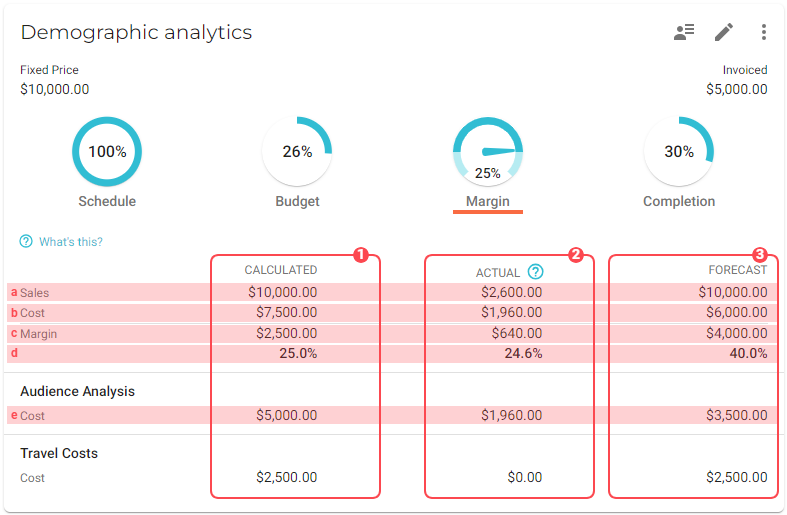# Margin when 'Fixed Price'

Taping on the margin indicator of a fixed price deliverable shows a detailed specification of the margin:Just like deliverables with invoicing type Time & Material, the specification of the margin shows the calculated margin (on the left) and the actual margin (on the right).

Also, the activities are listed where for each activity the calculated and actual costs is shown.

## Calculated margin

The calculated margin is based on the calculation of activities and the fixed price of the deliverable:

The calculated sales (labeled in the specification as ‘Fixed price’) is the fixed price value of the deliverable itself.

The calculated costs are based on the calculated activities of the deliverable. In this example there are two activities calculated:

Activity Calculated cost rate Hours calculated Total costs

Developing

\$100

\$200

\$20,000

Design

\$90

100

\$9,000

Total costs

The total costs per activity are also shown in the specification of the margin.

## Actual margin

The actual margin is based on actual costs and actual sales. Please find below how these are calculated:

### Actual costs

The calculation of actual costs for Fixed Price deliverables is exactly the same as ‘Time and material’.

The actual costs are based on all hours spent on the deliverable. The total number of hours spent is multiplied with by the cost rate of the employee who submitted the spent hours.

This example shows how the actual costs are calculated. In this situation, two employees tracked time on a fixed price deliverable:

Employee Cost rate employee Activity Hours spent Total costs

Mikey Johnson

\$90

Developing

32

\$2,880

William Smith

\$100

Design

12

\$1.200

\$4,080

### Actual sales

Since the revenue for fixed price deliverables is based by the invoice schedule, the total amount of all the billing periods is used for calculating the actual sales. To have a realistic actual sales value, VOGSY calculates the actual margin in proportion. How to determine the proportion can be configured in the Project settings function.

There are three options to choose from:

• Schedule: The percentage of the schedule is used. For example: If 25% of the time is passed, 25% of the total amount of the invoice schedule will be used.

• Budget: The percentage of budget spent is used. For example: when 30% of the budget is used, 30% of the total amount of the invoice schedule is used for calculating the actual margin.

• Completion: The percentage of completion determines the actual sales. The completion can be set manually by tapping on the completion indicator. When the deliverable has a completion percentage of 75%, the actual sales will be 75% of the total amount of the invoice schedule.

The default setting is ‘Completion’.

Based on the above example this would be the calculation of the actual margin, when the total amount of the invoicing schedule is \$37,500 and the completion is 15%:

 Actual costs Hours spent x cost rate employee \$4,080 Actual sales Invoicing schedule (\$37,500) x Completion (15%) \$5,625 Actual margin (\$) Actual sales - Actual costs \$1,545.00 Actual margin (%) Actual margin (\$) / Actual sales 27.5 %